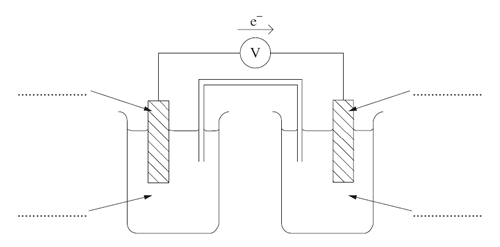Electrochemical cells (2009 NSW HSC) 1 )An electrochemical cell is constructed using two half cells. One half cell consists of an inert platinum electrode and a solution of Fe2+ and Fe3+. The other half cell consists of a lead electrode and a solution of Pb2+. Current will flow from one electrode to the other electrode when the cell is completed using a voltmeter and a salt bridge. (a) Write relevant half equations and a balanced net ionic equation for the overall cell reaction. Solution b) Calculate the standard cell potential (E° ). Solution c) Identify the anode, cathode, metals and ions by labelling the following diagram. Solutiond) Identify an appropriate electrolyte to use in the salt bridge. Solution